Next: An Illustration of Grover's Up: Quantum Computing and Grover's Previous: Performing Computations   Contents

# Grover's Algorithm

Assume you have a system with N = 2n states labeled S1, S2,...SN. These 2n states are represented by n bit strings. Assume there is a unique marked element Sm that satisfies a condition C(Sm) = 1, and for all other states C(S) = 0. We assume that C can be evaluated in unit time. Our task is to devise an algorithm which minimizes the number of evaluations of C.

The idea of Grover's algorithm is to place our register in a equal superposition of all states, and then selectively invert the phase of the marked state, and then perform an inversion about average operation a number of times. The selective inversion of the marked state follows by the inversion about average steps have the effect of increasing the amplitude of the marked state by O(1/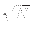). Therefore after O() operations the probability of measuring the marked state approaches 1. [Grover96]

Grover's algorithm is as follows:

1. Prepare a quantum register to be normalized and uniquely in the first state. Then place the register in an equal superposition of all states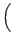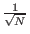,...by applying the Walsh-Hadamard operator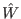. This means simply the state vector will be in an equal superposition of each state.

2. Repeat O() times the following two steps (the precise number of iterations is important, and discussed below):

1. Let the system be in any state S. If C(S) = 1, rotate the phase by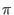radians, else leave system unaltered. It is worth noting that this operation has no classical analog. We do not observe the state of the quantum register, doing so would collapse the superposition. The selective phase rotation gate would be a quantum mechanical operator which would rotate only the amplitude proportional to the marked state within the superposition.

2. Apply the inversion about average operator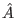, whose matrix representation is: Aij = 2/N if i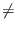j and Aii = - 1 + 2/N to the quantum register.

3. Measure the quantum register. The measurement will yield the n bit label of the marked state C(SM) = 1 with probability at least 1/2.

[Grover96]

SubsectionsNext: An Illustration of Grover's Up: Quantum Computing and Grover's Previous: Performing Computations   Contents
Matthew Hayward - Quantum Computing and Grover's Algorithm GitHub Repository